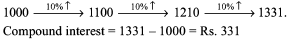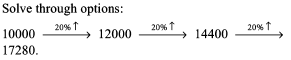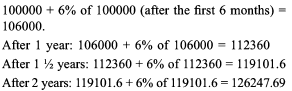Courses

# Test: SI & CI- 1

## 15 Questions MCQ Test Quantitative Aptitude (Quant) | Test: SI & CI- 1

Description
This mock test of Test: SI & CI- 1 for CAT helps you for every CAT entrance exam. This contains 15 Multiple Choice Questions for CAT Test: SI & CI- 1 (mcq) to study with solutions a complete question bank. The solved questions answers in this Test: SI & CI- 1 quiz give you a good mix of easy questions and tough questions. CAT students definitely take this Test: SI & CI- 1 exercise for a better result in the exam. You can find other Test: SI & CI- 1 extra questions, long questions & short questions for CAT on EduRev as well by searching above.
QUESTION: 1

### Rs. 1200 is lent out at 5% per annum simple interest for 3 years. Find the amount after 3 years.

Solution:

The annual interest would be Rs. 60. After 3 years the total value would be 1200 + 60 x 3 = 1380

QUESTION: 2

### Rs. 2100 is lent at compound interest of 5% per annum for 2 years. Find the amount after two years,

Solution:

2100 + 5% of 2100 = 2100 + 105 = 2205 (after 1 year). Next year it would become: 2205 + 5% of 2205 = 2205 +110.25 = 2315.25

QUESTION: 3

### Find the rate of interest if the amount after 2 years of simple interest on a capital of Rs. 1200 is Rs. 1440.

Solution:

Interest in 2 years = Rs. 240.
Interest per year = Rs. 120 Rate of interest = 10%

QUESTION: 4

What is the difference between the simple interest on a principal of Rs. 500 being calculated at 5% per annum for 3 years and 4% per annum for 4 years?

Solution:

5% for 3 years (SI) = 15% of the amount; At the same time 4% SI for 4 years means 16% of the amount. The difference between the two is 1% of the amount. 1% of 500 = Rs. 5

QUESTION: 5

What is the simple interest for 9 years on a sum of Rs. 800 if the rate of interest for the first 4 years is 8% per annum and for the last 4 years is 6% per annum?

Solution:

8% of 800 for 4 years + 6% of 800 for 4 years = 64 x 4 + 48 x 4 = 256 + 192 = 448. However, we do not know the rate of interest applicable in the 5 th year and hence cannot determine the exact simple interest for 9 years.

QUESTION: 6

Find the compound interest on Rs. 1000 at the rate of 20% per annum for 18 months when interest is compounded half-yearly.

Solution:QUESTION: 7

Find the principal if compound interest is charged on the principal at the rate of 162/3 % per annum for two years and the sum becomes Rs. 196.

Solution:

P x 7/6 x 7/6 = 196 → P = (196 x 6 x 6)/7 x 7 = 144.

QUESTION: 8

At what percentage per annum, will Rs. 10,000 amount to 17,280 in three years? (Compound Interest being reckoned)

Solution:QUESTION: 9

What is the rate of simple interest for the first 4 years if the sum of Rs. 360 becomes Rs. 540 in 9 years and the rate of interest for the last 5 years is 6%?

Solution:

For the last 5 years, the interest earned would be: 30% of 360 = 108. Thus, interest earned in the first 4 years would be Rs. 72 → Rs. 18 every year on an amount of Rs. 360- which means that the rate of interest is 5%

QUESTION: 10

Vinod makes a deposit of Rs. 100,000 in Syndicate Bank for a period of 2 years. If the rate of interest be 12% per annum compounded half-yearly, what amount will he get after 2 years?

Solution:QUESTION: 11

Ajay borrows Rs. 1500 from two moneylenders. He pays interest at the rate of 12% per annum for one loan and at the rate of 14% per annum for the other. The total interest he pays for the entire year is Rs. 186. How much does he borrow at the rate of 12%?

Solution:

The average rate of interest he pays is 186 x 100/1500 = 12.4%.

The average rate of interest being 12.4%, it means that the ratio in which the two amounts would be distributed would be 4:1 (using alligation). Thus, the borrowing at 12% would be Rs. 1200.

QUESTION: 12

The difference between simple and compound interest on a sum of money at 5% per annum is Rs. 25. What is the sum?

Solution:

The data is insufficient as we do not know the time period involved.

QUESTION: 13

Two equal sums were borrowed at 8% simple interest per annum for 2 years and 3 years respectively. The difference in the interest was Rs. 56. The sum borrowed were

Solution:

The difference would amount to 8% of the value borrowed. Thus 56 = 0.08 x sum borrowed in each case → Sum borrowed = Rs. 700.

QUESTION: 14

In what time will Rs. 500 give Rs. 50 as interest at the rate of 5% per annum simple interest?

Solution:

Interest per year = Rs. 25. Thus, an interest of Rs. 50 would be earned in 2 years.

QUESTION: 15

If the difference between the simple interest and compound interest on some principal amount at 20% per annum for 3 years is Rs. 48, then the principle amount must be

Solution:

Solve using options. If we try 500 (option b) for convenience, we can see that the difference between the two is Rs. 64 (as the SI would amount to 300 and Cl would amount to 100 + 120 + 144 = 364). Since, we need a difference of only Rs. 48 we can realize that the value should be 3/4th of 500. Hence, 375 is correct.